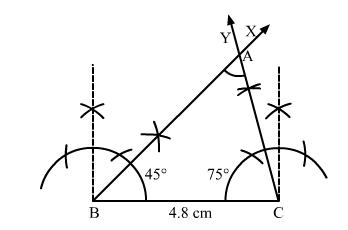# Construct a ΔABC in which BC = 4.8 cm,

Question:

Construct a ΔABC in which BC = 4.8 cm, ∠B = 45° and ∠C = 75°. Measure ∠A.

Solution:Steps of construction:

1. Draw a line segment BC = 4.8 cm.

2. Construct $\angle C B X=45^{\circ}$.

3. Construct $\angle B C Y=75^{\circ}$.

4. The ray $B X$ and $C Y$ intersect at $A$.

Thus, $\triangle A B C$ is the required triangle.

When we measure $\angle A$, we get $\angle A=60^{\circ}$.